Next: 13.3.3 Convolutions Using the Up: 13.3.2 The Convolution Operation Previous: 13.3.2.1 Two-Dimensional Convolutions   Contents

#### 13.3.2.2 Separable Filters

In the general case, the two-dimensional convolution operation requires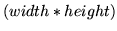multiplications for each output pixel. Separable filters are a special case of general convolution in which the filtercan be expressed in terms of two vectors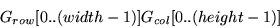such that for each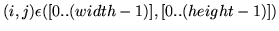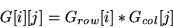If the filter is separable, the convolution operation may be performed using only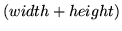multiplications for each output pixel. Applying the separable filter to Equation15 becomes: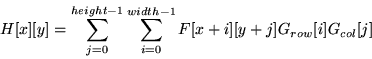Which can be simplified to: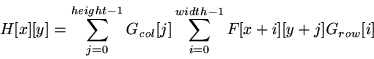To apply the separable convolution, first apply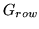as though it were a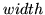by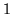filter. Then apply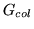as though it were aby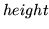filter.

2001-01-10